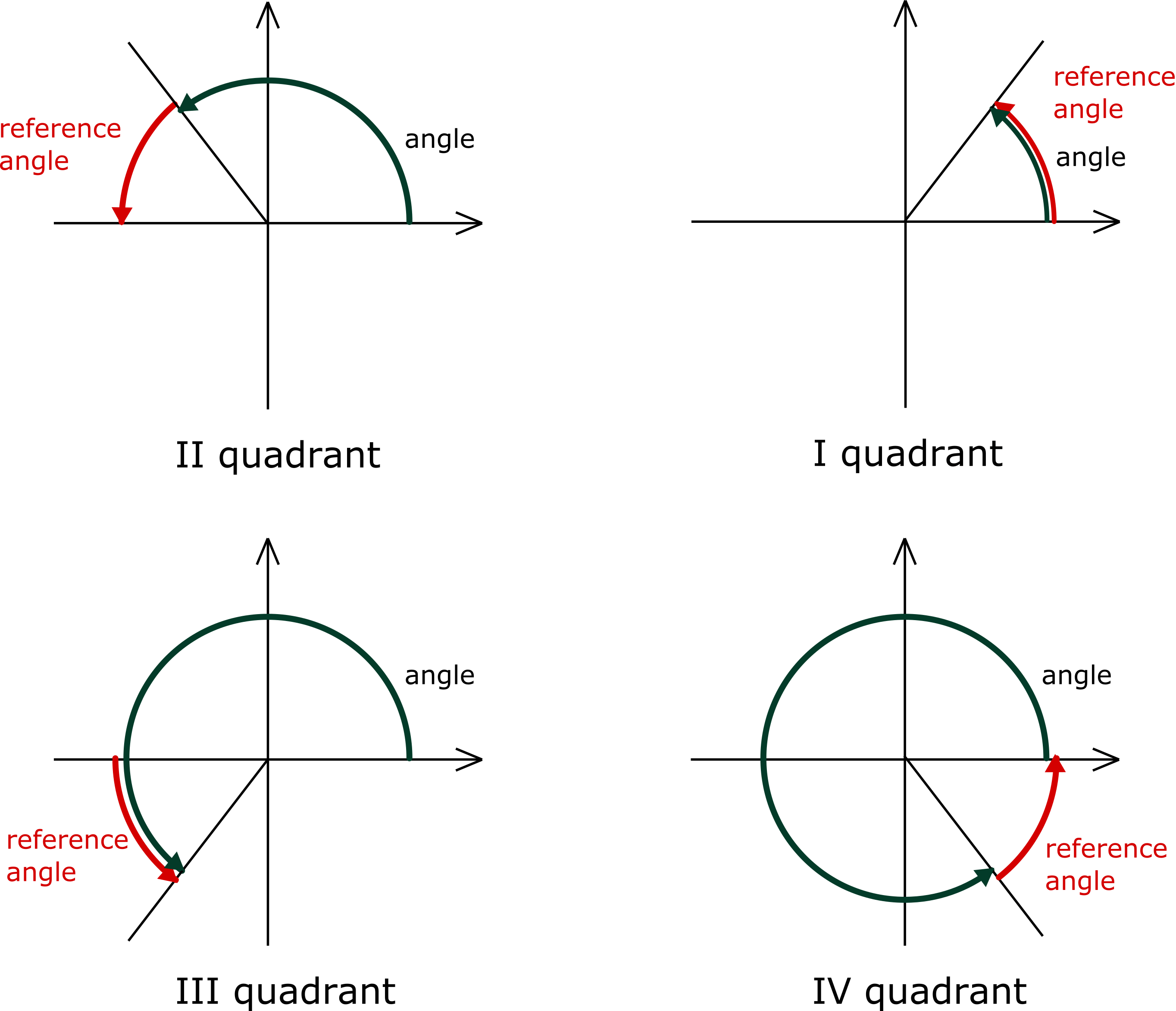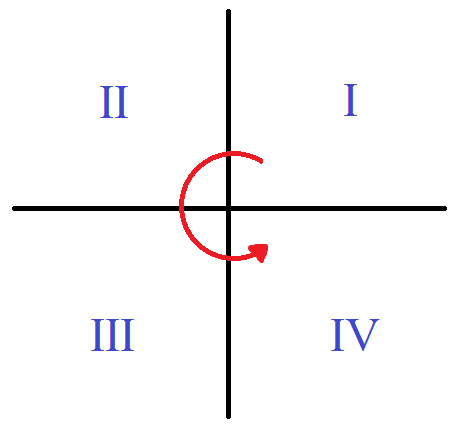# Reference Angle Calculator

Created by Bogna Szyk and Hanna Pamuła, PhD candidate
Reviewed by Steven Wooding and Jack Bowater
Last updated: Apr 12, 2022

Our reference angle calculator is a handy tool for recalculating angles into their acute version. All you have to do is simply input any positive angle into the field, and this calculator will find the reference angle for you. This article explains what a reference angle is, providing a reference angle definition.

It will also provide you with a step-by-step guide on how to find a reference angle in radians and degrees, along with a few examples. Keep scrolling, and you'll find a graph with quadrants as well!

## What is a reference angle? – Reference angle definitionLook at the picture above. Every angle is measured from the positive part of the x-axis to its terminal line (the line that determines the end of the angle) traveling counterclockwise. If you want to find the reference angle, you have to find the smallest possible angle formed by the x-axis and the terminal line, going either clockwise or counterclockwise.

Reference angles are useful in trigonometry. If you want to find the sine or cosine of any arbitrary angle, you first have to look for its reference angle in the first quarter. Then you can find the trigonometric function of the reference angle and choose a proper sign.

The most commonly used angles and their trigonometric functions can be found in the table below:

α(°) 30° 45° 60° 90° 180° 270° 360°
α(rad) 0 π/6 π/4 π/3 π/2 π 3π/2
sin(α) 0 1/2 √2/2 √3/2 1 0 −1 0
cos(α) 1 √3/2 √2/2 1/2 0 −1 0 1
tg(α) 0 √3/3 1 √3 - 0 - 0
ctg(α) - √3 1 √3/3 0 - 0 -

## Graph quadrants and trigonometric functionsThe two axes of a 2D Cartesian system divide the plane into four infinite regions called quadrants. Numbering starts from the upper right quadrant, where both coordinates are positive, and goes in an anti-clockwise direction, as in the picture.

Generally, trigonometric functions (sine, cosine, tangent, cotangent) give the same value for both an angle and its reference angle. The only thing that changes is the sign — these functions are positive and negative in various quadrants. Follow the "All Students Take Calculus" mnemonic rule (ASTC) to remember when these functions are positive.

• A for all: in the first quadrant, all trigonometric functions have positive values.
• S for sine: in the second quadrant, only the sine function has positive values.
• T for tangent: in the third quadrant, tangent and cotangent have positive values.
• C for cosine: in the fourth quadrant, only the cosine function has positive values.

If you don't like this rule, here are a few other mnemonics for you to remember:

• All Stations To Central 🚆
• All Science Teachers (are) Crazy 👩‍🏫

Make sure to take a look at our law of cosines calculator and our law of sines calculator for more information about trigonometry.

## How to find the reference angle for degrees?

All you have to do is follow these steps:

1. Choose your initial angle — for example, 610°.

2. If your angle is larger than 360° (a full angle), subtract 360°. Keep doing it until you get an angle smaller than a full angle. This is the same as finding the modulo. In this example, after subtracting 360°, we get 250°.

• 0° to 90° — first quadrant,
• 90° to 180° — second quadrant,
• 180° to 270° — third quadrant,
• 270° to 360° — fourth quadrant.

In this case, 250° lies in the third quadrant.

4. Choose the proper formula for calculating the reference angle:

• 0° to 90°: reference angle = angle,
• 90° to 180°: reference angle = 180° - angle,
• 180° to 270°: reference angle = angle - 180°,
• 270° to 360°: reference angle = 360° - angle.

In this case, we need to choose the formula reference angle = angle - 180°.

5. Substitute your angle into the equation to find the reference angle:

reference angle = 250° - 180° = 70°.

## How do I calculate the reference angle in radians?

It's easier than it looks!

1. For angles larger than 2π, subtract multiples of 2π until you are left with a value smaller than a full angle.
• 0 to π/2 — First quadrant, so reference angle = angle;
• π/2 to π — Second quadrant, so reference angle = π - angle;
• π to 3π/2 — Third quadrant, so reference angle = angle - π; and
• 3π/2 to 2π — Fourth quadrant, so reference angle = 2π - angle.

As you can see, this procedure is similar to the one above for degrees. As an example, let's consider

1. Having subtracted the multiples of 2π, we're left with 10π/9.
2. 10π/9 is a bit more than π, so it lies in the third quadrant. The reference angle is reference angle = angle - π = π/9.

## How to use this reference angle calculator?

It couldn't be easier! Simply:

1. Type the angle into the box. It may be any positive angle you imagine; let's say we want to find the reference angle for 210°. If you wish, you may also change the units, e.g., to radians.
2. That's it! This reference angle calculator found the acute version of your angle as well as the quadrant in which your initial angle lies. It's 30° in our case, and the initial angle lies in the third quadrant. Awesome!

## What is the reference angle for…

If you want a quick answer, have a look at the list below:

• Reference angle for 1°: 1°

• Reference angle for 2°: 2°

• Reference angle for 3°: 3°

• Reference angle for 4°: 4°

• Reference angle for 5°: 5°

• Reference angle for 6°: 6°

• Reference angle for 7°: 7°

• Reference angle for 8°: 8°

• Reference angle for 9°: 9°

• Reference angle for 10°: 10°

• Reference angle for 15°: 15°

• Reference angle for 20°: 20°

• Reference angle for 25°: 25°

• Reference angle for 30°: 30° (π / 6)

• Reference angle for 35°: 35°

• Reference angle for 40°: 40°

• Reference angle for 45°: 45° (π / 4)

• Reference angle for 50°: 50°

• Reference angle for 55°: 55°

• Reference angle for 60°: 60° (π / 3)

• Reference angle for 65°: 65°

• Reference angle for 70°: 70°

• Reference angle for 75°: 75°

• Reference angle for 80°: 80°

• Reference angle for 85°: 85°

• Reference angle for 90°: 90° (π / 2)

• Reference angle for 95°: 85°

• Reference angle for 100°: 80°

• Reference angle for 105°: 75°

• Reference angle for 110°: 70°

• Reference angle for 115°: 65°

• Reference angle for 120°: 60° (π / 3)

• Reference angle for 125°: 55°

• Reference angle for 130°: 50°

• Reference angle for 135°: 45° (π / 4)

• Reference angle for 140°: 40°

• Reference angle for 145°: 35°

• Reference angle for 150°: 30° (π / 6)

• Reference angle for 155°: 25°

• Reference angle for 160°: 20°

• Reference angle for 165°: 15°

• Reference angle for 170°: 10°

• Reference angle for 175°: 5°

• Reference angle for 180°: 0°

• Reference angle for 185°: 5°

• Reference angle for 190°: 10°

• Reference angle for 195°: 15°

• Reference angle for 200°: 20°

• Reference angle for 205°: 25°

• Reference angle for 210°: 30° (π / 6)

• Reference angle for 215°: 35°

• Reference angle for 220°: 40°

• Reference angle for 225°: 45° (π / 4)

• Reference angle for 230°: 50°

• Reference angle for 235°: 55°

• Reference angle for 240°: 60° (π / 3)

• Reference angle for 245°: 65°

• Reference angle for 250°: 70°

• Reference angle for 255°: 75°

• Reference angle for 260°: 80°

• Reference angle for 265°: 85°

• Reference angle for 270°: 90° (π / 2)

• Reference angle for 275°: 85°

• Reference angle for 280°: 80°

• Reference angle for 285°: 75°

• Reference angle for 290°: 70°

• Reference angle for 295°: 65°

• Reference angle for 300°: 60° (π / 3)

• Reference angle for 305°: 55°

• Reference angle for 310°: 50°

• Reference angle for 315°: 45° (π / 4)

• Reference angle for 320°: 40°

• Reference angle for 325°: 35°

• Reference angle for 330°: 30° (π / 6)

• Reference angle for 335°: 25°

• Reference angle for 340°: 20°

• Reference angle for 345°: 15°

• Reference angle for 350°: 10°

• Reference angle for 355°: 5°

• Reference angle for 360°: 0°

## FAQ

### Does a reference angle always exist?

Yes, each angle has a reference angle. For angles in the first quadrant, i.e., angles less than or equal to 90 degrees, the reference angle is equal to the original angle.

### What is the reference angle for 2pi?

The reference angle for 2π is 0. This is because 2π is the same as 0 radians in terms of reference angle, which is in the first quadrant. As such, the references angle is equal to the angle, and is therefore 0.

### What is the reference angle for 4pi over 3?

The reference angle for 4π/3 is π/3. 4π/3 is equivalent to 240°, which is in the third quadrant of a circle. As such, the reference angle is equal to the angle minus π, which in this case is π/3.

Bogna Szyk and Hanna Pamuła, PhD candidate
Angle
deg
Reference angle
deg
People also viewed…

### Car crash force

With this car crash calculator, you can find out how dangerous are car crashes.

### Coffee kick

A long night of studying? Or maybe you're on a deadline? The coffee kick calculator will tell you when and how much caffeine you need to stay alert after not sleeping enough 😀☕ Check out the graph below!

### Graphing inequalities on a number line

The graphing inequalities on a number line calculator allows up to three inequalities on one picture, and even returns what numbers satisfy all of them.

### Sum of Series

The sum of series calculator helps you find the sum of an arithmetic or geometric series. Using this tool, you can also find the sum of an infinite geometric series.